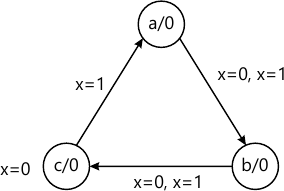MORE IN Design of Programming with Logic
VTU Computer Science (Semester 3)
Design of Programming with Logic
December 2013
Total marks: --
Total time: --
INSTRUCTIONS
(1) Assume appropriate data and state your reasons
(2) Marks are given to the right of every question
(3) Draw neat diagrams wherever necessary

1 (a) With the aid of circuit diagram, explain the operation of 2-input TTL NAND gate.
8 M
1 (b) What are universal gates? Implement the following function using universal gates only ((A+B⋅ C)D.
6 M
1 (c) Write the truth table of the logic circuit having 3 input A, B, and C and output expressed as y=ABC+ABC. Also simplify the expression using Boolean expression and implement the logic circuit using NAND gates?
6 M

2 (a) Using Q-M method, simplify the expression f(A, B, C, D)=∑ (0,3,5,6,7,11,14). Write the gate diagram for the simplified equation using NAND-NAND gates.
10 M
2 (b) A digital system is to be designed in which the month of the year given as input is four bit form. The month January is represented as '0000' February '0001' and so on. The output of the system should be '1' corresponding to the input of the month containing 31 days or otherwise it is '0' consider the excess number in the input beyond '1011' as don't care conditions for system of four variables (A,B,C,D) find the following:
i) Boolean expression in ∑m and πm form
ii) Write the truth table
iii) Using K-map, simplify the Boolean expression of canonical minterm form
iv) Implement the simplified equation using NAND-NAND gates.
10 M

3 (a) Write a 4:1 MUX verilog program using conditional 'assign' and 'case' statement.
6 M
3 (b) What are static hazards? How to design a hazard free circuit? Explain with an example.
6 M
3 (c) Explain IV-bit magnitude comparator.
8 M

4 (a) Draw the logic diagram of clock D-flip/flop write its truth table and characteristics equation, state diagram and excitation table, what is the draw back JK flip/flop.
10 M
4 (b) Differentiate between combinational circuit and sequential circuits.
5 M
4 (c) Show how a SR flip/flop can be conveterd into T-flip/flop.
5 M

5 (a) Using negative edge triggered JK flip/flop, draw the logic diagram of a 4-bit serial-in-serial-out shift register, Draw the waveform to shift the binary number 1010 into this register. Also draw the waveform for 4 clock transistor when J=K=0.
8 M
5 (b) Explain the working of mod-4 ring counter.
6 M
5 (c) Explain with a neat diagram, how shift register can be applied for serial addition.
6 M

6 (a) With the help of neat block diagram and timing diagram, explain the working of a Mod-16 ripple counter constructed using positive edge triggered JK flip-flops.
8 M
6 (b) Design asynchronous counter for the sequence 0 → 4 → 1 → 2 → 6 → 0 → 4. using SR flip-flop.
12 M

7 (a) Design a sequence detector that receives binary data stream as its input. X and signals when a combination '011' arrives at the input by making its output. Y high which otherwise remain line consider data is coming from left. i.e. the first bit to be indentified is 1. Second 1 and third 0 from the input sequence. Design mealy model?
14 M
7 (b) Realize the sequential circuit for the state diagram.6 M

8 (a) Explain 2-bit simultaneous A/D converter.
10 M
8 (b) What is binary ladder? Explain the binary ladder with a digital input of 1000.
6 M
8 (c) What is accuracy and resolution of the D/A converter? What is the resolution of a 12-bit D/A converter which uses binary ladder? If the full scale output is +10V. What is the resolution in volts?
4 M

More question papers from Design of Programming with Logic